# PI Function in Excel

Excel function that returns the value of the mathematical constant pi

The Pi(π) function in Excel returns the mathematical constant pi value, which is equal to 3.14159265358979.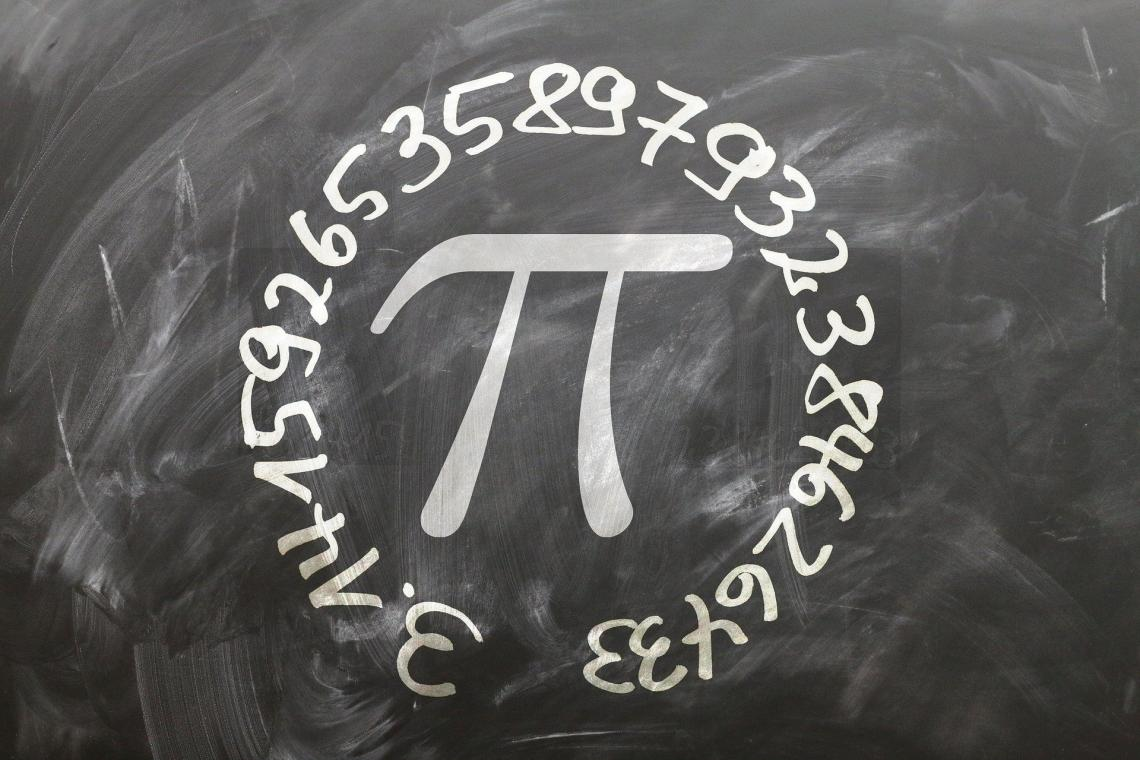Pi is one of the most well-known mathematical constants that has puzzled mathematicians for almost 4000 years.

The first time you are introduced to the concept of π in school is when you are expected to calculate the circumference of a circle using the value of Pi(π) and the diameter.

So suppose that if the circle's diameter is 10 cm and its circumference is 31.4 cm, what is the value of π?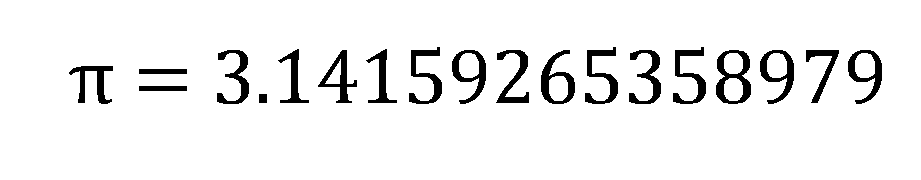We are aware that the formula for calculation of the circumference of the circle is:

Circumference = π x d

or

Circumference = 2 x π x r

where,

r = radius of the circle

d = diameter of the circle

By rearranging the formula, we get the value of π as:

π = (circumference/ diameter)

= 31.4 / 10

=3.14

From the calculations, we can deduce that π is the circle's circumference ratio to its diameter. Since circumference and diameter/radius are used in the same unit, π is just a number that does not carry any dimensions.

## Pi function - What is it?

It is categorized as a Math & Trigonometric function that returns the dimensionless value of the mathematical constant, π, equalling 3.14159265358979 (it is accurate up to 15 digits).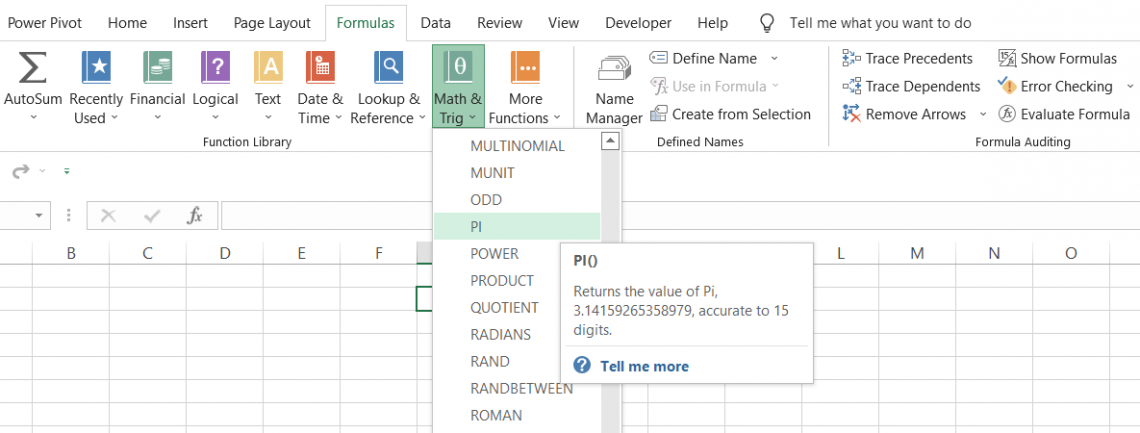Being an irrational number, Pi has indefinite recurring digits after the decimal. If you're curious, you can see the first 100,000 here. The human brain can't remember all the digits, and a computer can't store them all either, since there are infinite digits.

The best Excel does maintain accuracy up to the first 15 digits in various real-life calculations, such as for buildings and other architectural structures, quantum physics, medical procedures, and the trajectory of an aircraft in space.

Here's a great article on how NASA uses the value of Pi in its space adventures.

## Syntax for the function

The syntax for the function is:

=PI()

Where you don't need to add any additional arguments, you begin with the equal sign(=), followed by the function name, and end the formula with parenthesis or the round brackets. That's it!

So if you use the formula =PI() in Excel, the result that will be displayed in the spreadsheet will be: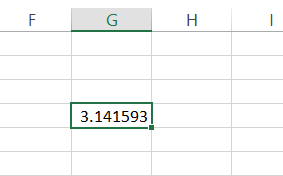Even though the function returns 14 digits after the decimal point, you can see that only six digits are represented in our spreadsheet.

You can either resize the cell's width or increase decimal points using the keyboard shortcut of Alt + H + 0 to improve the precision of the value.

Alternatively, you can also select the cell and press Ctrl +1. This will open up the Format cell dialog box where you can set the number of decimal places according to the required result's precision.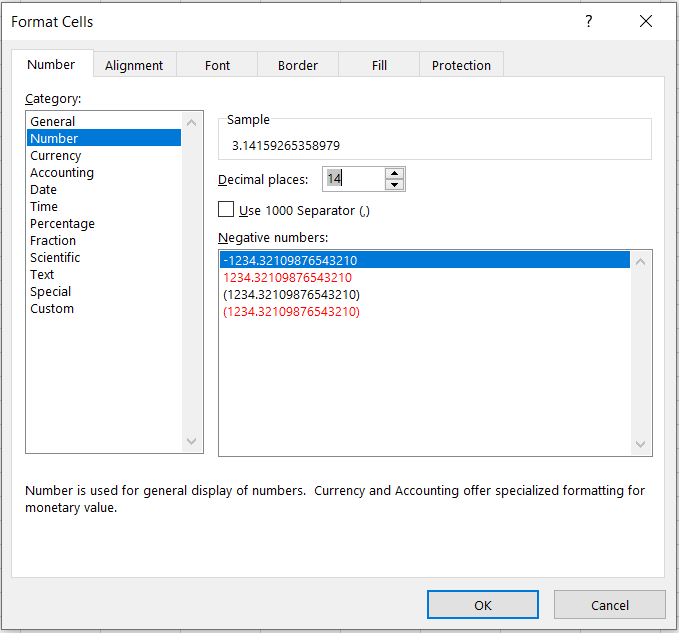You might notice that once the upper limit of 14 digits is crossed, Excel returns zero for all the decimal increases.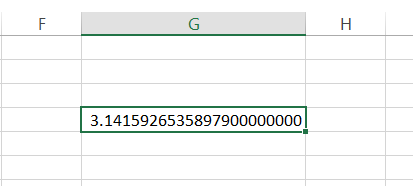## Example

There are different instances where you can use this function in Excel. You can use the function while calculating the:

1) Circumference of a Circle

Assume that you need to find the circumference of a circle with a diameter of 15 m. Then, the data in Excel looks as illustrated below:To calculate the circle's circumference, you will use the formula π x diameter or 2 x π x radius. You can either reference the value of π from another cell or directly input it into the formula.

Let's say we reference the value of π from cell C2. But, first, we will use the function =PI() in cell C2 so that we will get the result: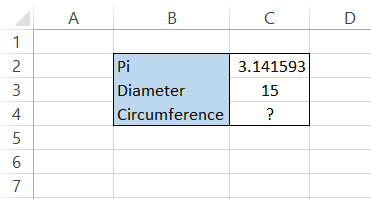Next, you need to do simple multiplication using the formula C2 x C3 in cell C4 to get the circle's circumference as 47.12389 m.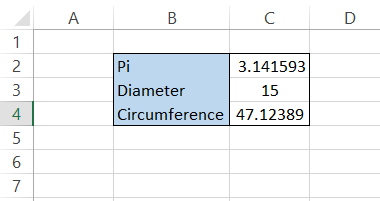Another method to calculate the circumference is to input the PI function in the calculations directly.

For example, we will use the formula =C3*PI() in cell C4, giving us the same result as the alternate method.

Note: If the result obtained has many digits after the decimal point, you can use the ROUND function and limit the decimals to 2 digits. For example, our formula becomes =ROUND(C3*PI(),2) to give the result as 47.12 m.

2) Area of a Circle

Let's say you need to calculate the circle's area with a diameter of 15 m. The formula to calculate the area of the circle is π x radius2. This situation is illustrated below: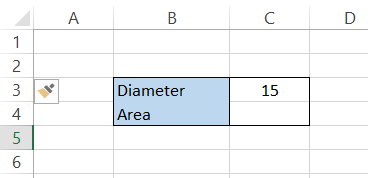First, we find the circle's radius, which is half the diameter. Therefore, the radius in cell C2 will be =C3/2.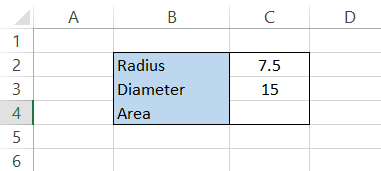Next, we will use the formula =PI()*C2^2, which will give the area of the circle as 176.71 m2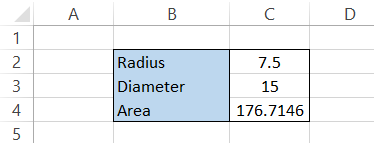Note: The caret (^) symbol is used to represent the power of a number in mathematics. Here, it is interpreted as the radius to the power of 2, i.e.radius2.

3) Volume of a sphere

The formula for the volume of a sphere is 4/3 x π x radius3. Assuming that the radius of the circle is 15m, the data is illustrated below: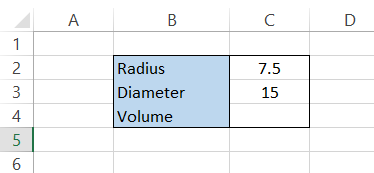Here, the radius equals =C3/2, giving the result 7.5m. The formula to calculate the volume of the sphere in cell C4 will be =4/3*PI()*(C2^3) to provide you with the result of 1767.146 m3

The function can convert the degrees into their radian counterparts. Radians are the standard international (SI) units used to measure angles instead of degrees.

When an angle of one radian is drawn from the center of the circle, it produces an arc length of 1. The angle that forms is approximately equal to 57 degrees or 1 radian. One degree is equal to (π/180) radians

Suppose you need to represent the degrees illustrated below into radians: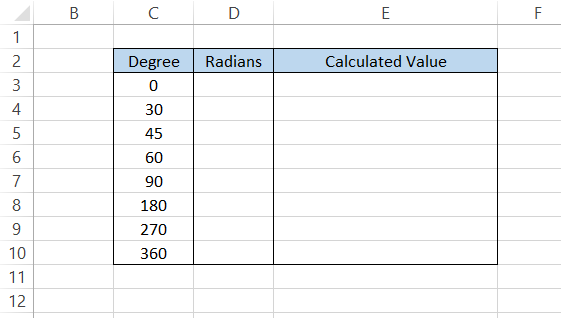So, if we need to change 30 degrees into radians, the formula will be 30 x (π /180), giving you the result as π/6 (the simplest form of the ratio).

Next, we substitute the value of π, i.e., 3.14159265, using the formula, =PI()/6, which will give you the result as 0.523598776 in cell E4.

Depending on the value in the denominator, the value of radian for its degree counterpart will be: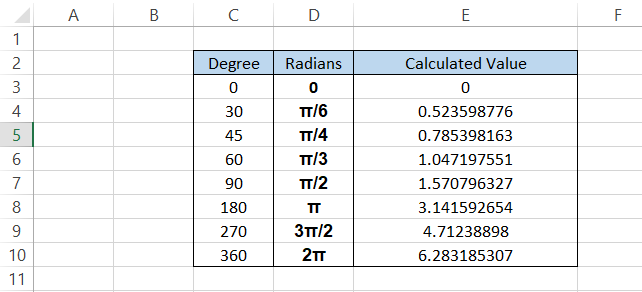As you can see, the magnitude of one complete revolution of the circle is equal to 2π, which is equal to 2 x 3.14159265, equalling 6.283185307

You can also directly use the formula =C3*PI()/180 in cell E3 and drag it down to the last cell, giving you the same result in the spreadsheet.

Once you understand how radians and degrees work in Excel, you can use the SIN, COS, and TAN trigonometric functions to derive their values.

For example, let's say you need to find the value of sin 90°. For this, we will use the formula =SIN(PI()/2), i.e., SIN(π/2), which will give us the result as 1.

Similarly, you can find the rest of the values by building a table as shown below: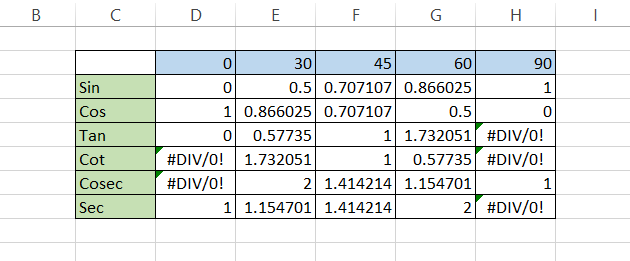For sin 30°, the result 0.5 can also be represented as ½. Similarly, sin 45° can be represented as 1/2. The formula used to find sin values is equal to =SIN(D2*(PI()/180)), while for cos and tan values it is =COS(D2*(PI()/180)) and =D3/D4 respectively.

The cot, cosec, and sec functions are the inverses of tan, sin, and cos, respectively (for example, cot = 1/tan)

## Practical Example #1

Let's say an architect needs financial advice from you regarding the construction of a dome-shaped structure on top of a tower.

The two options would cost \$480,000 and \$520,000, depending on the size and material (marble, granite, limestone, etc.) used for the dome construction.

The data is illustrated below: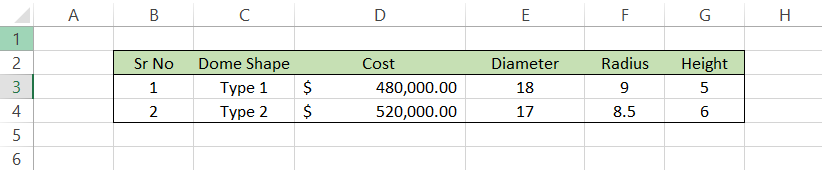Based on the inputs of both Type-1- and Type-2-shaped domes, the surface area of the dome can be calculated using the formula =ROUND(2*PI()*F3*G3,2), which will give us the result as: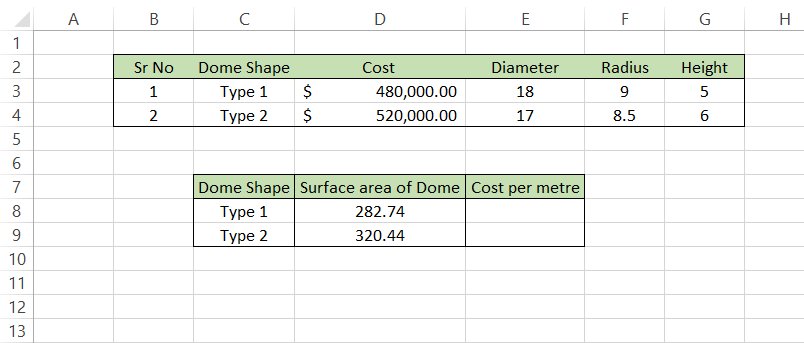The surface area of the Type 2 dome is equal to 320.44 m2 which is 37.7 m2 larger than Type 1 dome of 282.74 m2. Finally, we will divide the cost of construction of these domes by the surface area to get the cost per m2. We will use the formula  =D3/D8 in the spreadsheet to get the result: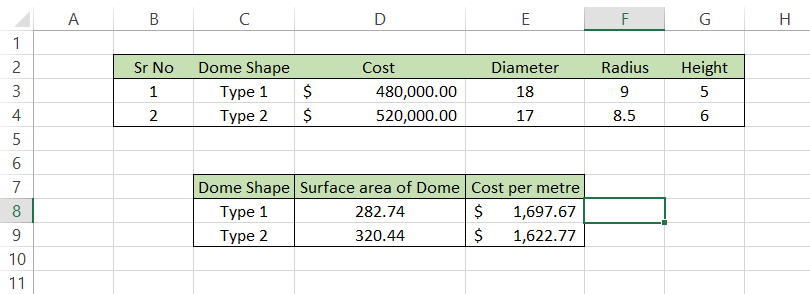So, by opting for the construction of dome Type 2, the architect can build a structure bigger than the one he would have built with a Type 1.

If the architect does not need to build a bigger structure, they can substitute the material used for the Type 1 dome and reduce the overall cost.

Important things to remember
• You will get the #NAME? Error if you forget to use the parenthesis or the round brackets following =PI in Excel.

• If you need to input the π symbol in Excel or Word, you can use the shortcut Alt + 227.

Researched and Authored by Akash Bagul | Linkedin

Reviewed and edited by James Fazeli-Sinaki | LinkedIn